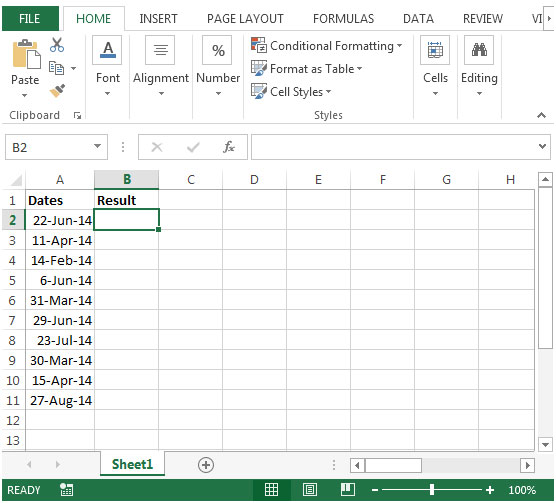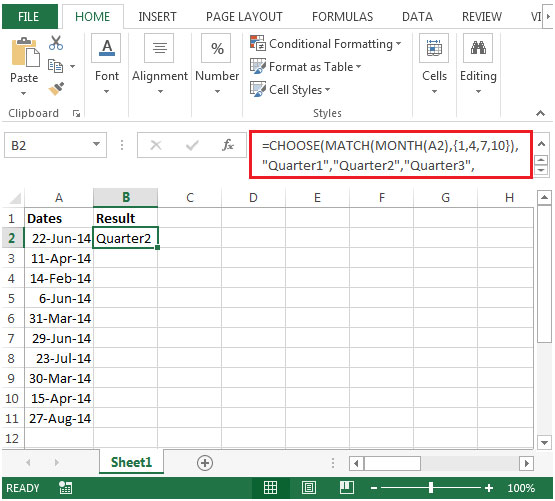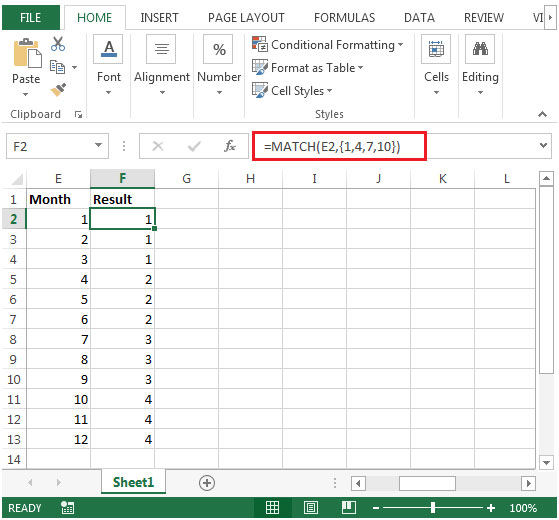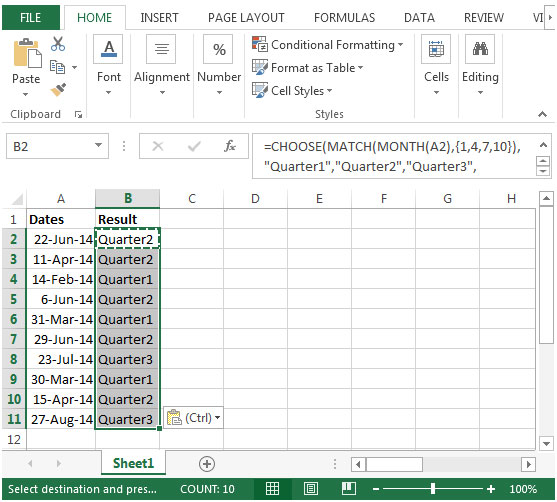# Determining which Quarter a Specified Date Corresponds To in Excel 2010

In this article, we will learn how to determine in which quarter a specified date corresponds.

While working on reports, you have some dates & you want a formula which will return the date as Quarter number of the current year. Example: Jan 1, 2014 to be return as Quarter 1.

We will use a combination of CHOOSE, MONTH & MATCH functions together to make a formula that will return the date in a cell into Quarter number.

Choose: Returns the character specified by the code number from the character set for your computer. CHOOSE function will return a value from a list of values based on a given index number. Choose function uses index_num to return a value from a list.

Syntax = CHOOSE(index_num,value1,value2,...)

index_num: It specifies which value argument is selected. Index_num must be a number between 1 and 254 or a formula that contains number between 1 and 254. If index_num is less than 1, then Choose will return #VALUE!error.

value1 & value 2 are 1 to 254 value arguments from which CHOOSE will evaluate & return the result.

MONTH: This function returns the month (January to December as 1 to 12) of a date.

Syntax: =MONTH(serial_number)

serial_number: It refers to the date of the month that you are trying to find.

MATCH function searches for a specified item in a selected range of cells, and then returns the relative position of that item in the range.

Syntax =MATCH(lookup_value,lookup_array,match_type)

lookup_value: The value you want to look for

lookup_array: The table of data contains information from which you want to return the output.

match_type: 1,0 and -1 are three options.

1(Default): It will find the largest value in the range. List must be sorted in ascending order.

0: It will find an exact match

-1: It will find the smallest value in the range. List must be sorted in descending order.

Let us take an example:

• We have some dates in column A
• In column B, we want a formula to return the Quarter number corresponding to date value in column A• The formula would be in cell B2=CHOOSE(MATCH(MONTH(A2),{1,4,7,10}),"Quarter1","Quarter2","Quarter3","Quarter4")• If we evaluate the MONTH(A2), then we will get 6 as result because month of June occurs 6th in the year.
• Then result returned by Month function will be then passed to Match function as its first argument as lookup value.
• If we evaluate the MATCH(MONTH(A2),{1,4,7,10})Match function will lookup the value of 6 (return by Month function) in the range of {1,4,7,10} and will return 2 because number 6 is less than 7.
• For more understanding, enter month numbers in column from 1 to 12 & then enter formula as =MATCH(E2,{1,4,7,10}). Refer below snapshot.• After evaluating the Match & Month formula, we will get CHOOSE(2,"Quarter1","Quarter2","Quarter3","Quarter4").
• If we further evaluate, CHOOSE function will check value 2 & return Quarter2 as our output.
• Copying down the same formula to range B2:B11, we will get the desired result1.